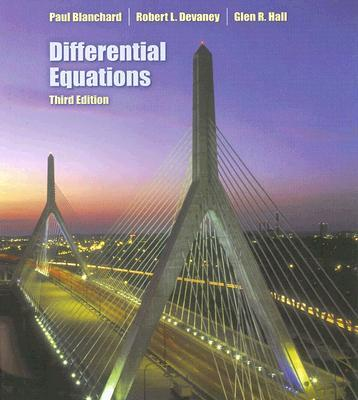### Differential Equations, second edition, by Blanchard, Devaney, and Hall. Published by Brooks/Cole Publishing Co. (a division of Thomson Learning), 2002. ISBN 0-534-38514-1.

Chapter 1. First-Order Differential Equations
• 1.1 Modeling Via Differential Equations
• 1.2 Analytic Technique: Separation of Variables
• 1.3 Qualitative Technique: Slope Fields
• 1.4 Numerical Technique: Euler's Method
• 1.5 Existence and Uniqueness of Solutions
• 1.6 Equilibria and the Phase Line
• 1.7 Bifurcations
• 1.8 Linear Differential Equations
• 1.9 Changing Variables
• Labs for Chapter 1

Chapter 2. First-Order Systems

• 2.1 Modeling via Systems
• 2.2 The Geometry of Systems
• 2.3 Analytic Methods for Special Systems
• 2.4 Euler's Method for Systems
• 2.5 The Lorenz Equations
• Labs for Chapter 2

Chapter 3. Linear Systems

• 3.1 Properties of Linear Systems and the Linearity Principle
• 3.2 Straight-Line Solutions
• 3.3 Phase Planes for Linear Systems with Real Eigenvalues
• 3.4 Complex Eigenvalues
• 3.5 Special Cases: Repeated and Zero Eigenvalues
• 3.6 Second-Order Linear Equations
• 3.7 The Trace-Determinant Plane
• 3.8 Linear Systems in Three Dimensions
• Labs for Chapter 3

Chapter 4. Forcing and Resonance

• 4.1 Forced Harmonic Oscillators
• 4.2 Sinusoidal Forcing
• 4.3 Undamped Forcing and Resonance
• 4.4 Amplitude and Phase of the Steady State
• 4.5 The Tacoma Narrows Bridge
• Labs for Chapter 4

Chapter 5. Nonlinear Systems

• 5.1 Equilibrium Point Analysis
• 5.2 Qualitative Analysis
• 5.3 Hamiltonian Systems
• 5.4 Dissipative Systems
• 5.5 Nonlinear Systems in Three Dimensions
• 5.6 Periodic Forcing of Nonlinear Systems and CHaos
• Labs for Chapter 5

Chapter 6. Laplace Transforms

• 6.1 Laplace Transforms
• 6.2 Discontinuous Functions
• 6.3 Second-Order Equations
• 6.4 Delta Functions and Impulse Forcing
• 6.5 Convolutions
• 6.6 The Qualitative Theory of Laplace Transforms
• Labs for Chapter 6

Chapter 7. Numerical Methods

• 7.1 Numerical Error in Euler's Method
• 7.2 Improving Euler's Method
• 7.3 The Runge-Kutta Method
• 7.4 The Effects of Finite Arithmetic
• Labs for Chapter 7

Chapter 8. Discrete Dynamical Systems

• 8.1 The Discrete Logistic Equation
• 8.2 Fixed and Periodic Points
• 8.3 Bifurcations
• 8.4 Chaos
• 8.5 Chaos in the Lorenz System
• Labs for Chapter 8